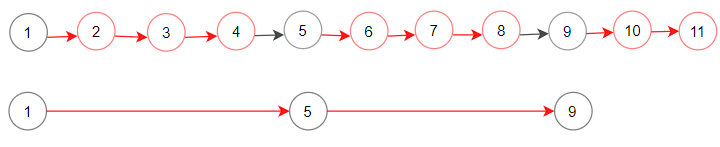# 1474. Delete N Nodes After M Nodes of a Linked List

Given the `head` of a linked list and two integers `m` and `n`. Traverse the linked list and remove some nodes in the following way:

• Keep the first `m` nodes starting with the current node.
• Remove the next `n` nodes
• Keep repeating steps 2 and 3 until you reach the end of the list.

Return the head of the modified list after removing the mentioned nodes.

Follow up question: How can you solve this problem by modifying the list in-place?

Example 1:```Input: head = [1,2,3,4,5,6,7,8,9,10,11,12,13], m = 2, n = 3
Output: [1,2,6,7,11,12]
Explanation: Keep the first (m = 2) nodes starting from the head of the linked List  (1 ->2) show in black nodes.
Delete the next (n = 3) nodes (3 -> 4 -> 5) show in read nodes.
Continue with the same procedure until reaching the tail of the Linked List.

Example 2:```Input: head = [1,2,3,4,5,6,7,8,9,10,11], m = 1, n = 3
Output: [1,5,9]

Example 3:

```Input: head = [1,2,3,4,5,6,7,8,9,10,11], m = 3, n = 1
Output: [1,2,3,5,6,7,9,10,11]
```

Example 4:

```Input: head = [9,3,7,7,9,10,8,2], m = 1, n = 2
Output: [9,7,8]
```

Constraints:

• The given linked list will contain between `1` and `10^4` nodes.
• The value of each node in the linked list will be in the range` [1, 10^6]`.
• `1 <= m,n <= 1000`

Easy

Prime

Microsoft

### Solution(Chinese):

LEETCODE 1474. Delete N Nodes After M Nodes of a Linked List 解题思路分析